# Github Repository 可视化 (D3.js & Three.js)

### Github Repository 可视化 (D3.js & Three.js)

demo 链接
github 链接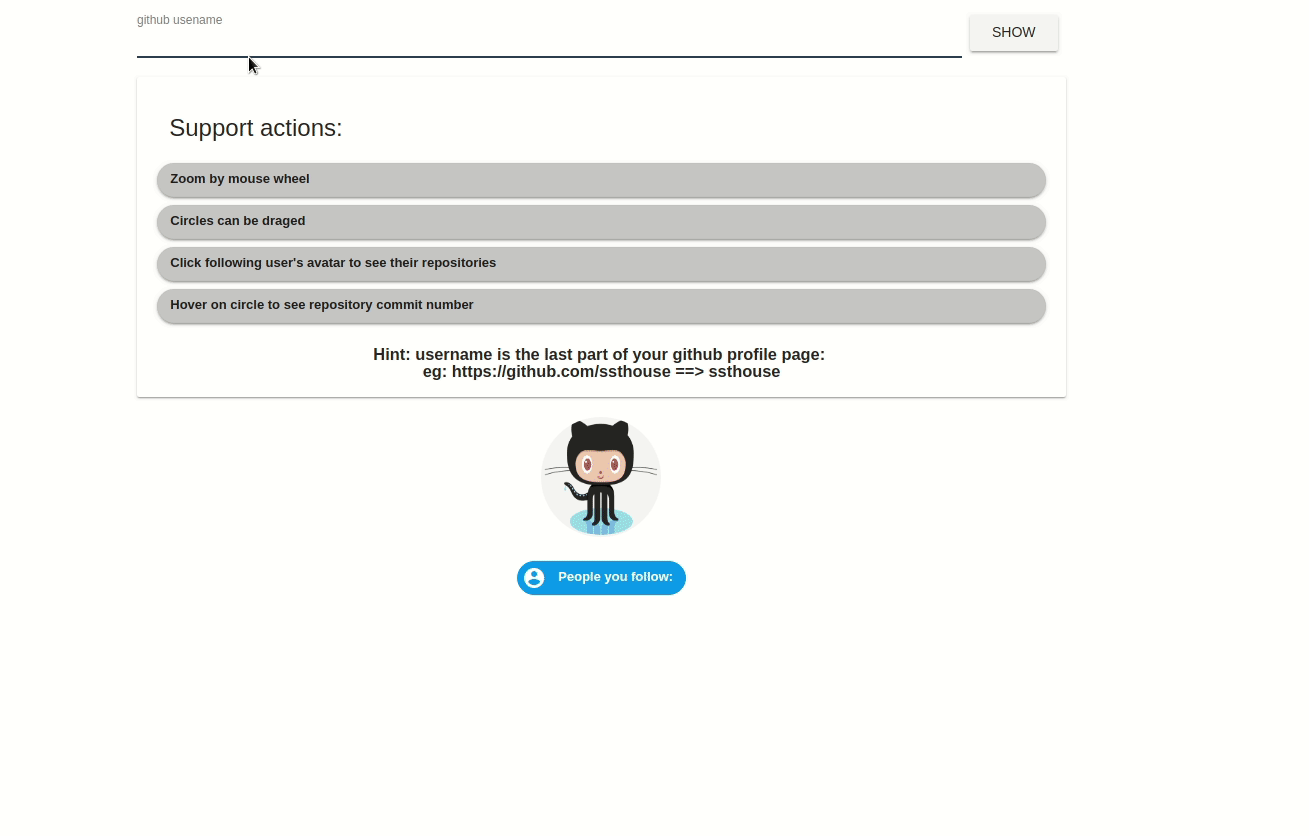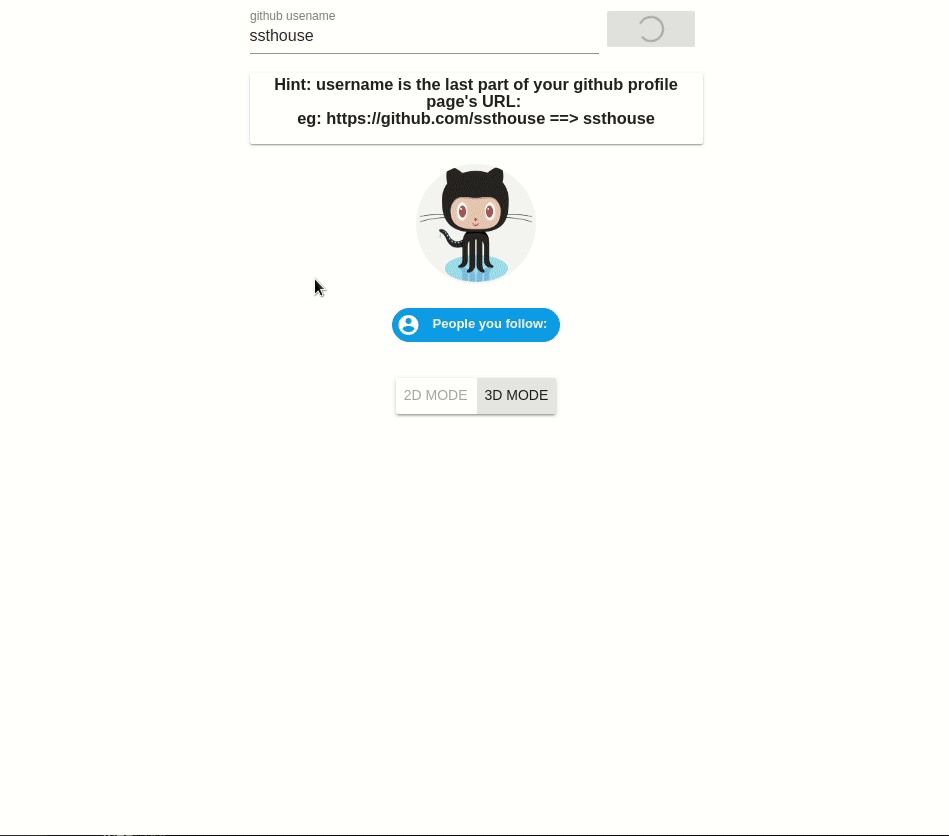### 实现的功能有哪些?

2D 和 3D 版本均支持:

• 展示用户的 Repository 可视化效果
• 点击 following people 的头像查看他人的 Repository 可视化效果

### 用到了哪些技术?

• 数据来源为 Github 提供的 GraphQL API.
• 2D 实现使用到了 D3.js
• 3D 实现使用到了 Three.js
• 页面搭建使用 Vue.js

### 实现细节?

#### 2D 实现

2D 效果图中, 每一个 Repository 用一个圆形表示, 圆形的大小代表了 _commit 数目 || start 数目 || fork 数目_.

==> 检测鼠标拖拽事件

==> 更新 UI 元素坐标

==> 重新计算布局坐标

==> 更新 UI 来达到圆形可拖拽的效果.

##### 让我们来看看具体代码:

2D 页面依赖 D3.js 的 force-layout 进行动态更新, 我们为 force-layout 添加了以下几种 force(作用力):

• .force('charge', this.$d3.forceManyBody()) 添加节点之间的相互作用力 • .force('collide',radius) 添加物理碰撞, 半径设置为圆形的半径 • .force('forceX', this.$d3.forceX(this.width / 2).strength(0.05)) 添加横坐标居中的作用力
• .force('forceY', this.$d3.forceY(this.height / 2).strength(0.05)) 添加纵坐标居中的作用力 主要代码如下: this.simulation = this.$d3
.forceSimulation(this.filteredRepositoryList)
.force('charge', this.$d3.forceManyBody()) .force( 'collide', this.$d3.forceCollide().radius(d => this.areaScale(d.count) + 3)
)
.force('forceX', this.$d3.forceX(this.width / 2).strength(0.05)) .force('forceY', this.$d3.forceY(this.height / 2).strength(0.05))
.on('tick', tick)

const tick = function() {
const curTransform = self.$d3.zoomTransform(self.div) self.updateTextLocation() const texts = self.div.selectAll('span').data(self.filteredRepositoryList) texts .enter() .append('span') .merge(texts) .text(d => d.name) .style('font-size', d => self.textScale(d.count) + 'px') .style( 'left', d => d.x + self.width / 2 - ((self.areaScale(d.count) * 1.5) / 2.0) * curTransform.k + 'px' ) .style( 'top', d => d.y - (self.textScale(d.count) / 2.0) * curTransform.k + 'px' ) .style('width', d => self.areaScale(d.count) * 1.5 + 'px') texts.exit().remove() const repositoryCircles = self.g .selectAll('circle') .data(self.filteredRepositoryList) repositoryCircles .enter() .append('circle') .append('title') .text(d => 'commit number: ' + d.count) .merge(repositoryCircles) .attr('cx', d => d.x + self.width / 2) .attr('cy', d => d.y) .attr('r', d => self.areaScale(d.count)) .style('opacity', d => self.alphaScale(d.count)) .call(self.enableDragFunc()) repositoryCircles.exit().remove() } 完成以上的逻辑后, 就能看到 2D 初始加载数据时的效果了: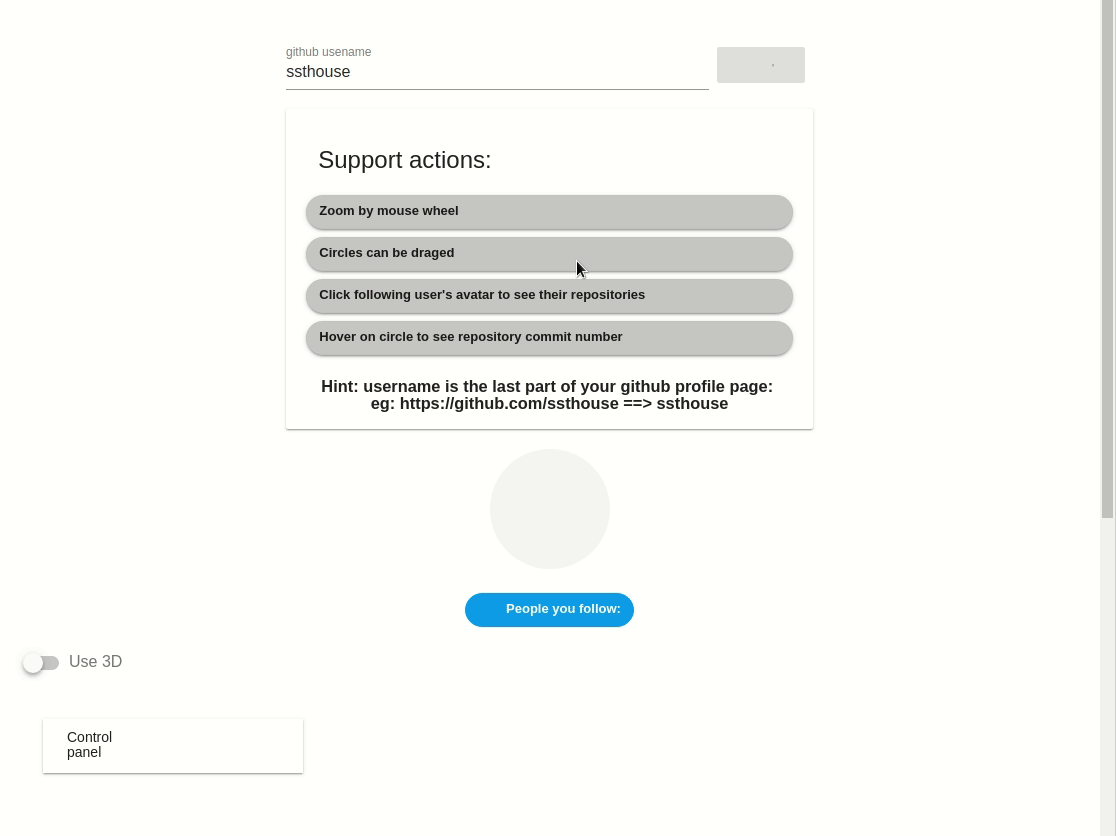但此时页面中的 圆圈 (circle)还不能响应鼠标拖拽事件, 让我们使用 d3-drag 加入鼠标拖拽功能. 代码非常简单, 使用 d3-drag 处理 start, drag, end 三个鼠标事件的回调即可: • start & drag ==> 将当前节点的 fx, fy (即 forceX, forceY, 设置这两个值会让 force-layout 添加作用力将该节点移动到 fx, fy) • end ==> 拖拽事件结束, 清空选中节点的 fx, fy, enableDragFunc() { const self = this this.updateTextLocation = function() { self.div .selectAll('span') .data(self.repositoryList) .each(function(d) { const node = self.$d3.select(this)
const x = node.style('left')
const y = node.style('top')
node.style('transform-origin', '-' + x + ' -' + y)
})
}
return this.$d3 .drag() .on('start', d => { if (!this.$d3.event.active) this.simulation.alphaTarget(0.3).restart()
d.fx = this.$d3.event.x d.fy = this.$d3.event.y
})
.on('drag', d => {
d.fx = this.$d3.event.x d.fy = this.$d3.event.y
self.updateTextLocation()
})
.on('end', d => {
if (!this.$d3.event.active) this.simulation.alphaTarget(0) d.fx = null d.fy = null }) }, 需要注意的是,我们在 drag 的回调方法中,调用了 updateTextLocation(), 这是因为我们的 drag 事件将会被应用到 circle 上, 而 text 不会自动更新坐标, 所以需要我们去手动更新. 接下来,我们将 d3-drag 应用到 circle 上: const repositoryCircles = self.g .selectAll('circle') .data(self.filteredRepositoryList) repositoryCircles .enter() .append('circle') .append('title') .text(d => 'commit number: ' + d.count) .merge(repositoryCircles) .attr('cx', d => d.x + self.width / 2) .attr('cy', d => d.y) .attr('r', d => self.areaScale(d.count)) .style('opacity', d => self.alphaScale(d.count)) .call(self.enableDragFunc()) // add d3-drag function repositoryCircles.exit().remove() 如此我们便实现了拖拽效果: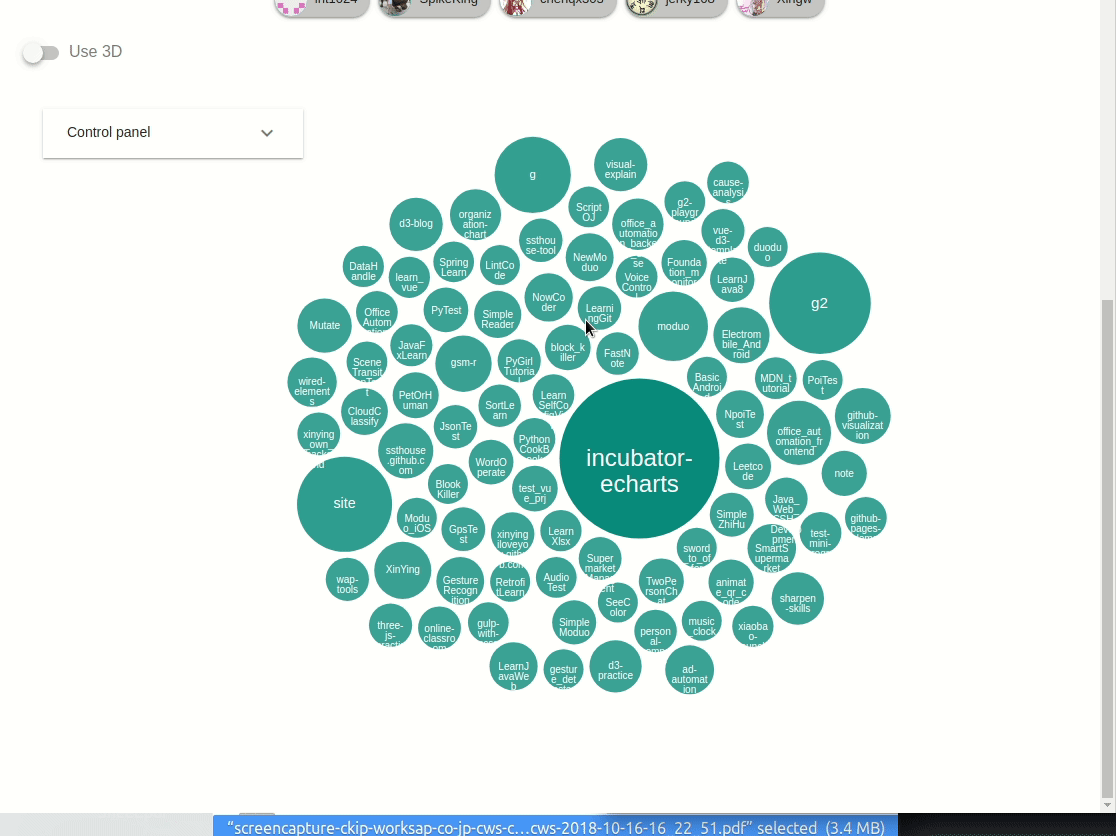最后让我们加上 2D 界面的缩放功能, 这里使用的是 d3-zoom. 和 d3-drag 类似, 我们只用处理鼠标滚轮缩放的回调事件即可: enableZoomFunc() { const self = this this.zoomFunc = this.$d3
.zoom()
.scaleExtent([0.5, 10])
.on('zoom', function() {
self.g.attr('transform', self.$d3.event.transform) self.div .selectAll('span') .data(self.repositoryList) .each(function(d) { const node = self.$d3.select(this)
const x = node.style('left')
const y = node.style('top')
node.style('transform-origin', '-' + x + ' -' + y)
})
self.div
.selectAll('span')
.data(self.repositoryList)
.style(
'transform',
'translate(' +
self.$d3.event.transform.x + 'px,' + self.$d3.event.transform.y +
'px) scale(' +
self.\$d3.event.transform.k +
')'
)
})
this.g.call(this.zoomFunc)
}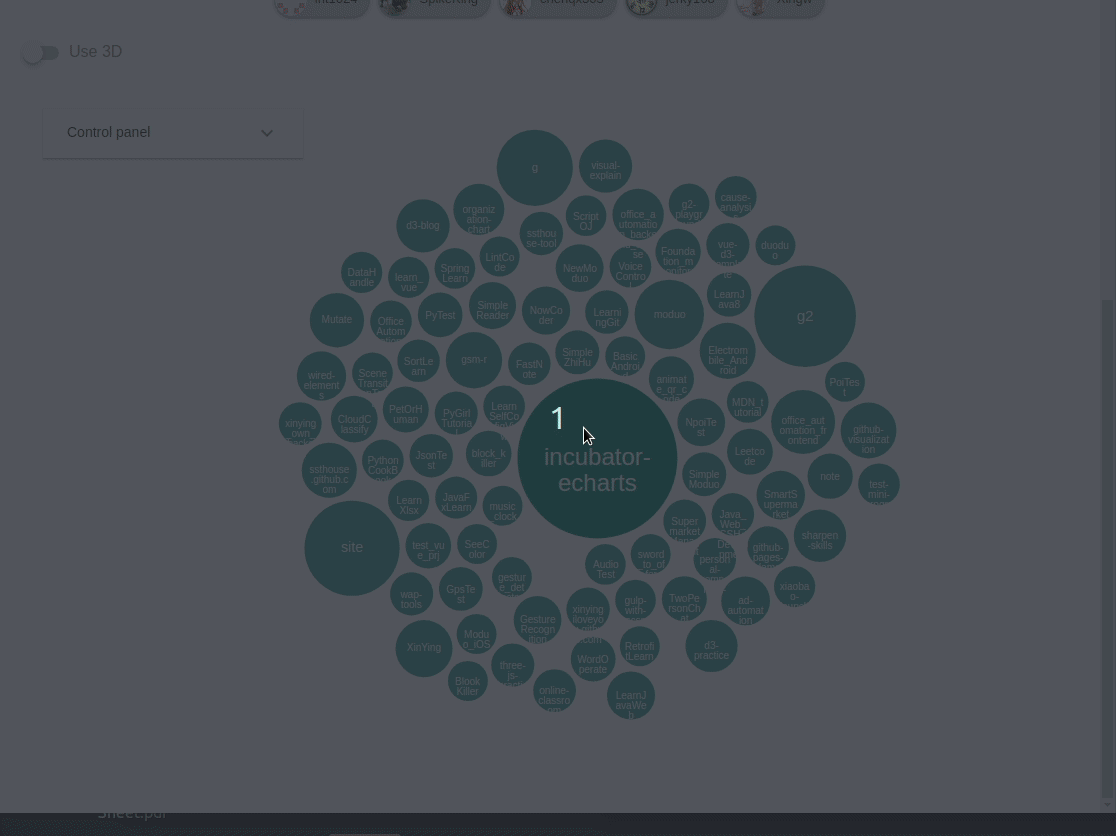#### 3D 实现

3D 效果图中的布局使用的是 d3-layout 中的 pack layout, 3D 场景中的拖拽合缩放直接使用了插件 three-orbit-controls.

##### 创建基本 3D 场景

3D 视图中, 承载所有 UI 组件的是 Three.js 中的 Scene,首先我们初始化 Scene.

this.scene = new THREE.Scene()

this.renderer = new THREE.WebGLRenderer({ alpha: true, antialias: true })
this.renderer.setClearColor(0xeeeeee, 0.3)
var contaienrElement = document.getElementById(this.containerId)
contaienrElement.appendChild(this.renderer.domElement)

// add light
var light = new THREE.AmbientLight(0x404040, 1) // soft white light
this.scene.add(light)
var spotLight = new THREE.DirectionalLight(0xffffff, 0.7)
spotLight.position.set(0, 0, 200)
spotLight.lookAt(0, 0, 0)
this.scene.add(spotLight)

this.renderer.render(this.scene, this.camera)

  animate_() {
requestAnimationFrame(() => this.animate_())
this.controls.update()
this.renderer.render(this.scene, this.camera)
}
##### 实现布局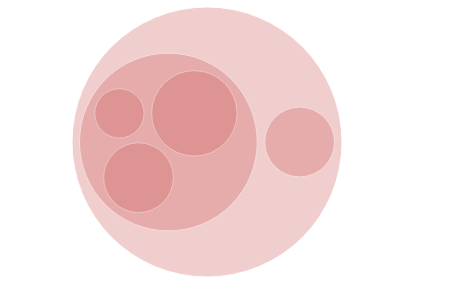{
"children": this.reporitoryList
}

calcluate3DLayout_() {
const pack = D3.pack()
.size([this.layoutSize, this.layoutSize])
.padding(5)
const rootData = D3.hierarchy({
children: this.reporitoryList
}).sum(d => Math.pow(d.count, 1 / 3))
this.data = pack(rootData).leaves()
}

##### 创建表示 Repository 的球体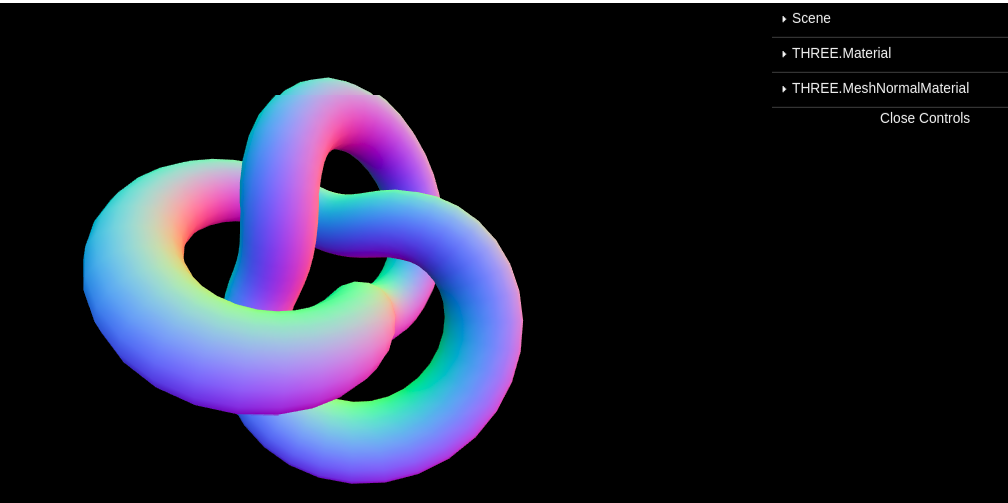addBallsToScene_() {
const self = this
if (!this.virtualElement) {
this.virtualElement = document.createElement('svg')
}
this.ballMaterial = new THREE.MeshNormalMaterial()
const circles = D3.select(this.virtualElement)
.selectAll('circle')
.data(this.data)
circles
.enter()
.merge(circles)
.each(function(d, i) {
const datum = D3.select(this).datum()
self.ballGroup.add(
self.generateBallMesh_(
self.indexScale(datum.x),
self.indexScale(datum.y),
self.volumeScale(datum.r),
i
)
)
})
}

generateBallMesh_(xIndex, yIndex, radius, name) {
var geometry = new THREE.SphereGeometry(radius, 32, 32)
var sphere = new THREE.Mesh(geometry, this.ballMaterial)
sphere.position.set(xIndex, yIndex, 0)
return sphere
}

##### 创建表示 Repository 名称的 文字物体

github issue: https://github.com/mrdoob/three.js/issues/1825

// 事先将26个字母创建好 TextGeometry
loadAlphabetGeoMap() {
const fontSize = 2.4
this.charGeoMap = new Map()
this.charWidthMap = new Map()
const chars =
'1234567890abcdefghijklmnopqrstuvwxyzABCDEFGHIJKLMNOPQRSTUVWXYZ_-./?'
chars.split('').forEach(char => {
const textGeo = new THREE.TextGeometry(char, {
font: this.font,
size: fontSize,
height: 0.04
})
textGeo.computeBoundingBox()
const width = textGeo.boundingBox.max.x - textGeo.boundingBox.min.x
this.charGeoMap.set(char, textGeo)
this.charWidthMap.set(char, width)
})
console.log(this.charGeoMap)
}

// 创建整个单词时直接使用现有字母的 TextGeometry进行拼接
addTextWithCharGroup(text, xIndex, yIndex, radius) {
const group = new THREE.Group()
const chars = text.split('')

let totalLen = 0
chars.forEach(char => {
if (!this.charWidthMap.get(char)) {
totalLen += 1
return
}
totalLen += this.charWidthMap.get(char)
})
const offset = totalLen / 2

for (let i = 0; i < chars.length; i++) {
const curCharGeo = this.charGeoMap.get(chars[i])
if (!curCharGeo) {
xIndex += 2
continue
}
const curMesh = new THREE.Mesh(curCharGeo, this.textMaterial)
curMesh.position.set(xIndex - offset, yIndex, radius + 2)
group.add(curMesh)
xIndex += this.charWidthMap.get(chars[i])
}
this.textGroup.add(group)
}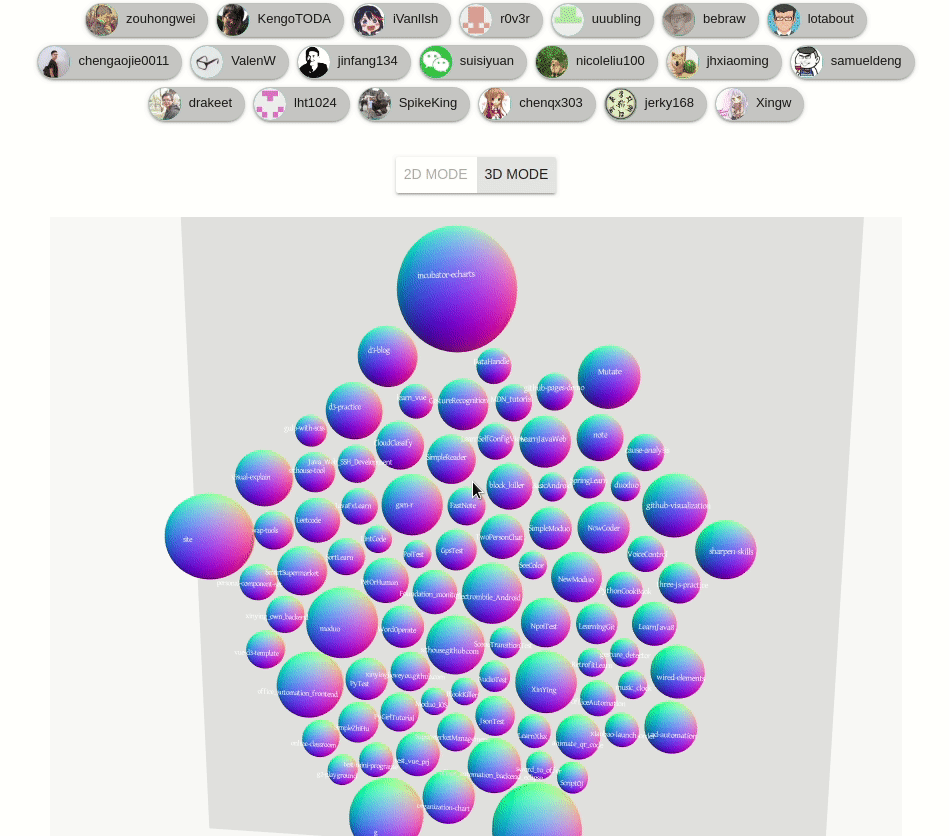D3-blog

## 如果觉得本文不错的话, 不妨点击下面的链接关注一下 : )

github 主页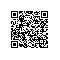使用钉钉扫一扫加入圈子
+ 订阅